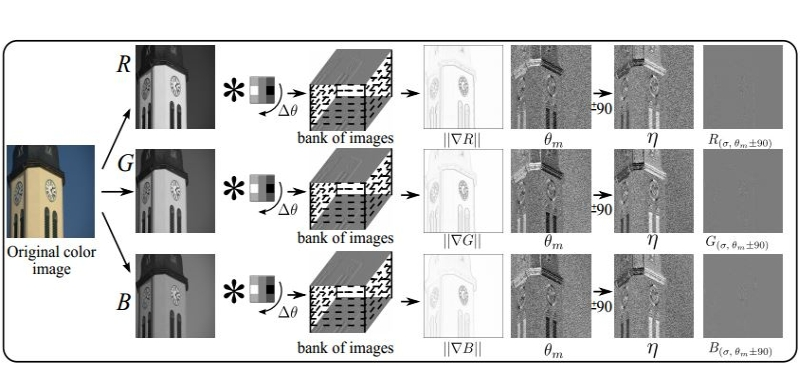# FAQ

... on the paper "Color Image Steganalysis Based on Steerable Gaussian Filters Bank,"

Hasan Abdulrahman, Marc Chaumont, Philippe Montesinos, and Baptiste Magnier, "Color Image Steganalysis Based on Steerable Gaussian Filters Bank," IH&MMSec'2016, in Proceedings of the 4th ACM workshop on Information Hiding and Multimedia Security, Vigo, Galicia, Spain, 6 pages, June 20-22, 2016. Acceptance Rate = 36.2%. pdfslides. Matlab code.

## About the dimension of the feature vector:

The first set, produced by , is made of 18,157 features.
The second set is made of 4406 features and comes from SPAM features from
- the gradient magnitude image of each channel (R, G, B) ; it gives 2808 features;
- the derivative image (related to the tangent vector) for each channel (R, G, B) ; it gives 1598 features.

More details about the second set:
- The co-occurences matrices are computed with triplets which means that co-occurences have (2xT+1)^3 bins.
- 4 matrices are computed (horizontal right, and left, and vertical right, and left) and then summed.
- 4 others matrices are computed (horizontal right, and left, and vertical right and left) and then summed.
- The two matrices are then concatenated in a feature vector.
- For each T values (except for the derivative image with T=3), the feature vectors of each channel  are concatenated.

Thus:
- For the gradient magnitude, T is equal to 2 or 3. This leads to a dimension = 3x(2x5^3) + 3x(2x7^3) = 3x250 + 3x686 = 2808 features,
- For the derivative image, T is equal to 1, 2 or 3. This leads to a dimension = 3x(2x3^3) + 3x(2x5^3) + (2x7^3) = 3x54+3x250+686 = 1598 features.
Note that for T=3, we sum the 3 co-occurrences matrices (from R, G, B channels) instead of concatenate them (indeed, otherwise, bins values are too small for T=3).